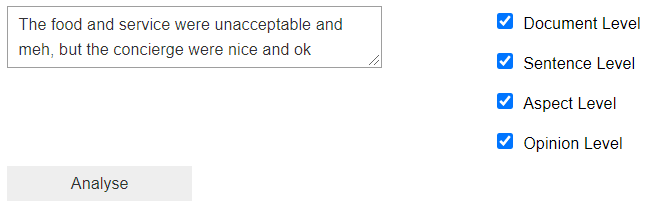# Opinion Mining - Aspect Level Sentiment Analysis

Aspect level sentiment analysis employs multiple machine learning processes. The first is parsing the sentence to extract the relation between words and be able to identify the aspects of a review. The second is analysing the sentiment of the adjectives used to describe the aspects.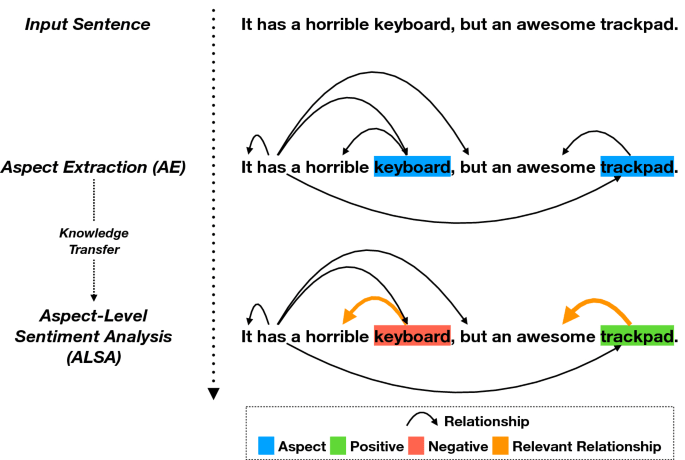This can be done automatically using Azure's Text Analytics service. All we need to do is to create a free account on microsoft azure and create a text analytics service: link

1. Once you create and login to your account go to azure portal.
2. Search for Text Analytics and create a new service.
3. It will ask for a resource group, click on "create new"
4. Choose the free tier which works fine for personal experimentation.
5. Once the service is created, go to your resources and look for Keys and Endpoints, copy the keys and put them in the following cell.
In :
KEY = "PUT THE KEY HERE"
ENDPOINT = "PUT THE ENDPOINT HERE"


This function is just header to authenticate your credentials and connect with Azure. We can the communicate with the Azure ML service through the client object.

In [ ]:
from azure.ai.textanalytics import TextAnalyticsClient
from azure.core.credentials import AzureKeyCredential

def authenticate_client():
ta_credential = AzureKeyCredential(KEY)
text_analytics_client = TextAnalyticsClient(
endpoint=ENDPOINT,
credential=ta_credential)
return text_analytics_client

client = authenticate_client() # we will interact with Azure ML via this object.


We will use Jupyter's widgets to create an interactive tool for opinion mining.

In :
import ipywidgets as widgets


We will use Plotly library for interactive visualizations.

In [ ]:
import plotly.graph_objs as go
from plotly.offline import init_notebook_mode
from plotly.subplots import make_subplots
init_notebook_mode() # this line is required to be able to export the notebook as html with the plots.

In :
# given three score (positive - neutral - negative) this function plots a pie chart of the three sentiments
def plot_sentiment_scores(pos, neut, neg):
return go.Figure(go.Pie(labels=["Positive", "Neutral", "Negative"], values=[pos, neut, neg],
textinfo='label+percent',
marker=dict(colors=["#2BAE66FF", "#795750", "#C70039"])),
layout=dict(showlegend=False)
)


## Sentiment Analysis using Azure's Text Analytics

Azure's Text analytics analyzes documents, not just sentences. Each document is a list of sentences. So our input must be a list of sentences.

We can use our Azure client to call the analyze_sentiment method, which will return a list of sentiment scores for each passed document. Since we are just using one document with one sentence, we are interested in the first thing it returns, which is a tuple of three values: positive, negative, and neutral sentiment scores.

In [ ]:
response = client.analyze_sentiment(documents=["This movie is fantastic"])
response

In [ ]:
response


AnalyzeSentimentResult(id=0, sentiment=positive, warnings=[], statistics=None, confidence_scores=SentimentConfidenceScores(positive=1.0, neutral=0.0, negative=0.0), sentences=[SentenceSentiment(text=This movie is fantastic, sentiment=positive, confidence_scores=SentimentConfidenceScores(positive=1.0, neutral=0.0, negative=0.0), offset=0, mined_opinions=[])], is_error=False)

In [ ]:
print(f"Positive: {response.confidence_scores.positive}")
print(f"Neutral: {response.confidence_scores.neutral}")
print(f"Negative: {response.confidence_scores.negative}")


Positive: 1.0
Neutral: 0.0
Negative: 0.0

Let's put all of this in a function that takes a list of sentences as an input and plots the distribution of sentiment scores as a pie chart!

In [ ]:
def sentiment_analysis_example(sentences):
document = [sentences] # we use only one document for this function
response = client.analyze_sentiment(documents=document) # we use  to get only the first and only document
print("Document Sentiment: {}".format(response.sentiment))
plot_sentiment_scores(response.confidence_scores.positive,
response.confidence_scores.neutral,
response.confidence_scores.negative
).show()

# here we plot the sentiment for each sentence in the document.
for idx, sentence in enumerate(response.sentences):
print("Sentence: {}".format(sentence.text))
print("Sentence {} sentiment: {}".format(idx+1, sentence.sentiment))
plot_sentiment_scores(sentence.confidence_scores.positive,
sentence.confidence_scores.neutral,
sentence.confidence_scores.negative
).show()

In [ ]:
sentiment_analysis_example("The acting was good. The graphics however were just okayish. I did not like the ending though.")


Document Sentiment: mixed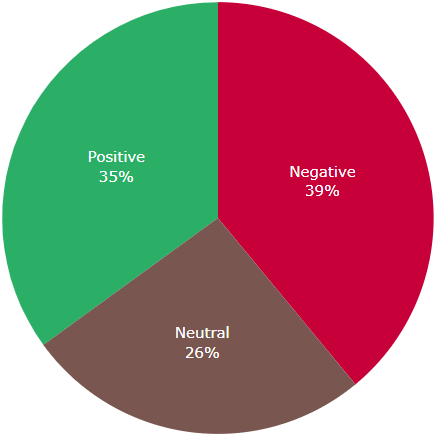Sentence: The acting was good.
Sentence 1 sentiment: positive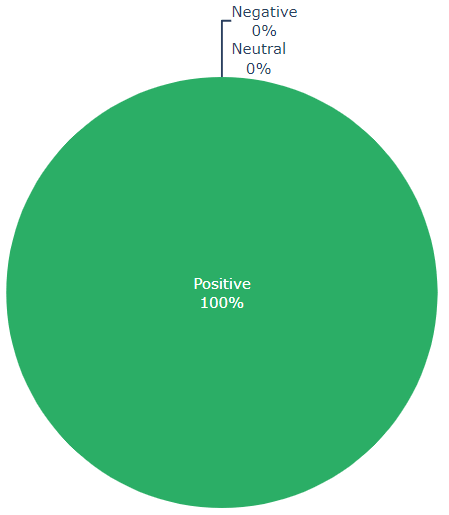Sentence: The graphics however were just okayish.
Sentence 2 sentiment: negative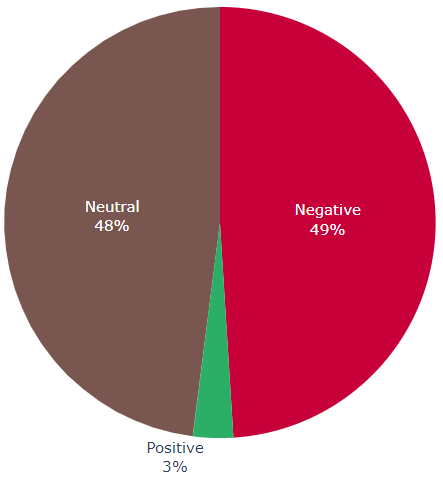Sentence: I did not like the ending though.
Sentence 3 sentiment: negative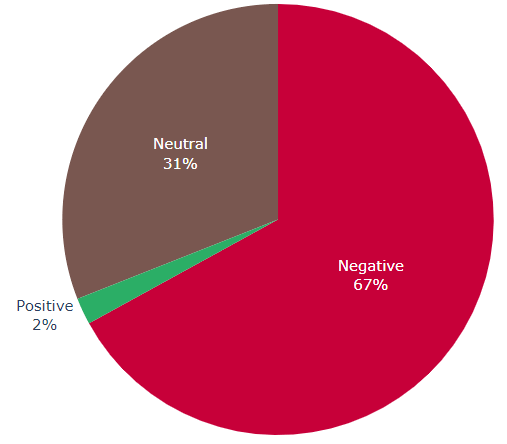## Aspect Level Opinion Mining Using Azure Text Analytics

Instead of just reporting the overall sentiment of a sentence, in aspect-level opinion mining, there are two main differences:

1. We extract specific aspects in the sentences.
2. We detect the opinion about the aspect in the text, not just a sentiment score.
In [ ]:
repsonse = client.analyze_sentiment(
["The food and service were unacceptable and meh, but the concierge were nice and ok"],
show_opinion_mining=True # only addition is that we set show_opinion_mining to True
)

In [ ]:
# now we can also access the mined_opinions in a sentence
mined_opinion = repsonse.sentences.mined_opinions
aspect = mined_opinion.aspect
print(f"Aspect: {aspect.text}")
for opinion in mined_opinion.opinions:
print(f"Opinion: {opinion.text}\tSentiment:{opinion.sentiment}".expandtabs(12))
# p.s. we use expandtabs because unacceptable is longer than 8 characters
# , so we want the \t to consider it one long word


Aspect: food Opinion:
unacceptable Sentiment:negative
Opinion: meh Sentiment:mixed

Let's make this more visual

In [ ]:
def plot_sentiment_gauge(pos_score, title, domain=[0, 1]):
fig = go.Figure(go.Indicator(
mode="gauge+number",
value=pos_score,
gauge={'axis': {'range': [0, 1]}},
domain={'x': domain, 'y': [0, 1]},
title={'text': f"{title}", "font":dict(size=14)}), layout=dict(width=800, height=600, margin=dict(l=150,r=150)))
return fig

In [ ]:
def sentiment_analysis_with_opinion_mining_example(sentences,
document_level=True,
sentence_level=True,
aspect_level=True,
opinion_level=True):

document = [sentences]

response = client.analyze_sentiment(document, show_opinion_mining=True)

if document_level:  # plotting overall document sentiment
print("Document Sentiment: {}".format(response.sentiment))
plot_sentiment_scores(response.confidence_scores.positive,
response.confidence_scores.neutral,
response.confidence_scores.negative
).show()
if not(sentence_level or aspect_level or opinion_level):
# no need to continue if no plots are needed
return response

for sentence in response.sentences:
if sentence_level:  # plotting the overall sentence sentiment
print(f"Sentence: {sentence.text}")
print(f"Sentence sentiment: {sentence.sentiment}")
plot_sentiment_scores(
sentence.confidence_scores.positive,
sentence.confidence_scores.neutral,
sentence.confidence_scores.negative).show()

for mined_opinion in sentence.mined_opinions:
aspect = mined_opinion.aspect

if aspect_level:  # plotting the sentiment of the aspect
plot_sentiment_gauge(
aspect.confidence_scores.positive, f"Aspect ({aspect.text})").show()

if opinion_level:
opinions = mined_opinion.opinions
n = len(opinions)
gauges = list()
for i, opinion in enumerate(opinions, start=1):
gauges.append(plot_sentiment_gauge(
opinion.confidence_scores.positive, f"Opinion ({opinion.text})",
# this is just to show the plots next to each other
domain=[(i-1)/n, i/n]
).data)

go.Figure(gauges, layout=go.Layout(
height=600, width=800, autosize=False)).show()
return response

In [ ]:
response = sentiment_analysis_with_opinion_mining_example(
"The food and service were unacceptable and meh, but the concierge were nice and ok",
document_level=False, sentence_level=False
)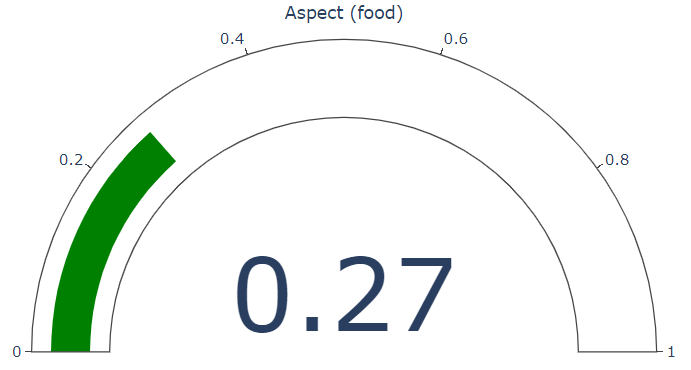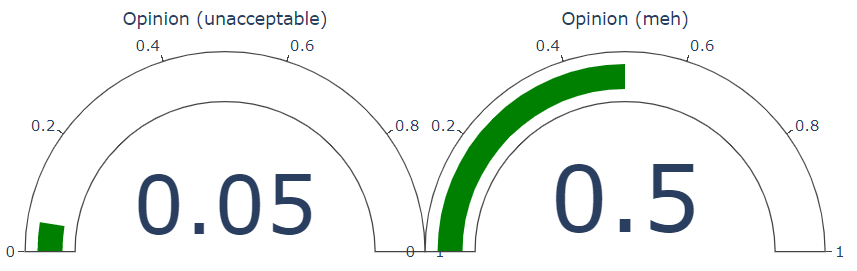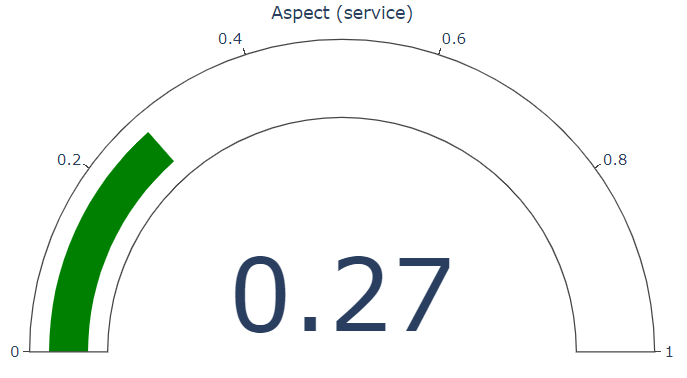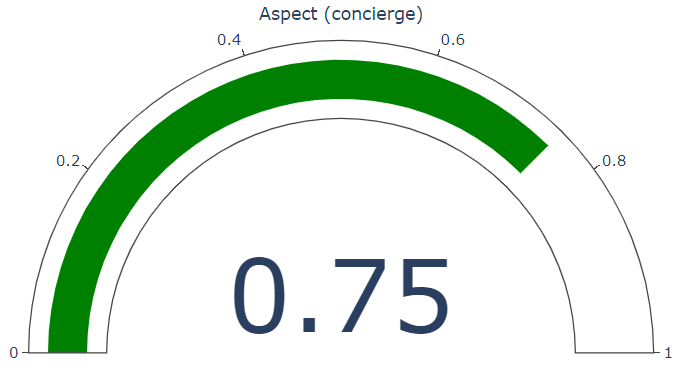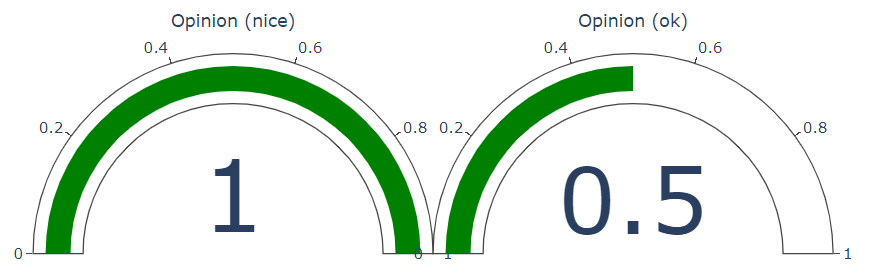## Text Analytics using Jupyter widgets

Now let's create some jupyter widgets to interact with this function.

In [ ]:
# some text to get the input
text = widgets.Textarea(placeholder="Enter your text here")
# checkboxes to select different levels of analysis
document_cb = widgets.Checkbox(value=True, description="Document Level")
sentence_cb = widgets.Checkbox(value=True, description="Sentence Level")
aspect_cb = widgets.Checkbox(value=True, description="Aspect Level")
opinion_cb = widgets.Checkbox(value=True, description="Opinion Level")

# some button to trigger the analysis
btn = widgets.Button(description="Analyse")

# some place to show the output on
out = widgets.Output()

def analysis(b):
with out:
out.clear_output()
sentences = text.value # get the input sentences from the Textarea widget
# pass the input sentences to our sentiment_analysis_example function
sentiment_analysis_with_opinion_mining_example(sentences,
document_level=document_cb.value,
sentence_level=sentence_cb.value,
aspect_level=aspect_cb.value,
opinion_level=opinion_cb.value
)

btn.on_click(analysis) # bind the button with the sentiment_analysis function

# put all widgets together in a tool
checkboxes = widgets.VBox([document_cb, sentence_cb, aspect_cb,opinion_cb])
tool = widgets.VBox([widgets.HBox([text, checkboxes]), btn, out])
# give a default value for the text
text.value = "The food and service were unacceptable and meh, but the concierge were nice and ok"
tool